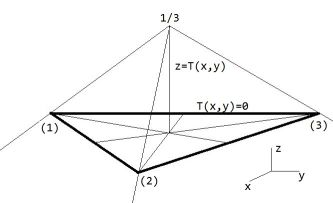## Is there any equation for triangle?

Some "closed" expressions for the triangle are quite useful, as is obvious from the following web page: Efficient 2-D & 3-D Point Probes.

The gist of the method is the so-called isoparametric ("same parameters") transformation, where "isoparametric" is a terminology which is quite common in Finite Element contexts. An elaborate explanation of it has been given, as an answer to the following MSE question: Converting triangles to isosceles, equilateral or right???

The formulas which express the local coordinates $\xi$ and $\eta$ into the global coordinates $x$ and $y$ are repeated here for convenience: $$\begin{array}{ll} \xi = [ (y_3 - y_1).(x - x_1) - (x_3 - x_1).(y - y_1) ]/\Delta \\ \eta = [ (x_2 - x_1).(y - y_1) - (y_2 - y_1).(x - x_1) ]/\Delta \end{array}$$ Here $\Delta$ is the determinant of the inverse transformation.
With the above, the "closed" triangle equation $T(x,y) = 0$ is simply given with: $$T(x,y) = \min( \xi , \eta , 1 - \xi - \eta )$$The maximum of the function $T$ is reached for $\xi = \eta = 1 - \xi - \eta = 1/3$ , hence at the midpoint (barycenter) of the triangle. If we draw straight lines from the midpoint towards the vertices, and further, then the whole plane is subdivided into three regions, one where $T(x,y) = \xi$ , one where $T(x,y) = \eta$ and one where $T(x,y) = 1 - \xi - \eta$ . Our "inside/outside" function $T$ is zero at the triangle sides, positive inside and negative outside. It's shaped like a mountain with top $1/3$ at the midpoint and three sharply edged slopes downhill. The contour lines of this function are triangles, where the contour line with height $0$ is the original triangle itself. ( Quite the same is the case with the equation of e.g. a circle: $C(x,y) = 0$ with $C(x,y) = R^2 - (x-a)^2 - (y-b)^2$. )

A generalization of the linear triangle in 2-D to a linear tetrahedron in 3-D is given in the following answer : Computing a three-dimensional Lebesgue measure of a bounded set .